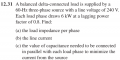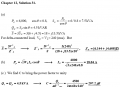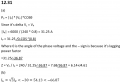# three phase delta load problem

#### skyline1397

Joined Sep 13, 2017
6
Hi all,
I have solved the problem attached and my answer isn't the same as the solution manual. Can you please review and advise me if I am wrong or not?
The problem is:The solution manual approach:My approach:#### Tech_Elect

Joined Feb 13, 2019
8
Hi,

it's a delta load so you have to multiply the formula of active power P by root of 3 because the delta load is subjected to line voltage.

for me the whole procedure of the text is correct.

in fact, phase current = line current (in this case for delta load)
(from what I could understand by reading the text)*

18.04 A (line current)

the line current leads the phase current of 30 ° angle

Regards

#### Tech_Elect

Joined Feb 13, 2019
8
Hi,

it's a delta load so you have to multiply the formula of active power P by root of 3 because the delta load is subjected to line voltage.
P=√3*VL*IL*cosθ

for me the whole procedure of the text is correct.

in fact, phase current = line current (in this case for delta load)
***it's a typo
correction: phase current= line current (in wye load)
phase current = IL/√ 3 (in delta load)

18.04 A (line current)

the line current leads the phase current of 30 ° angle
***correction: the line current lags the phase current of 30° angle (balanced load)

Regards